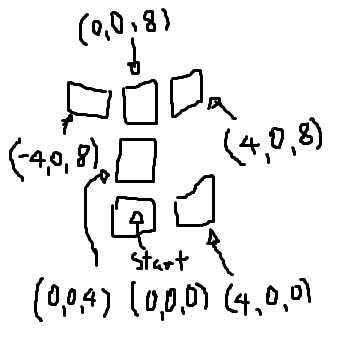# Rotation Snapping is adding harmful floats!

Here’s the story,

So my game is set up so that there are tiled platforms set up into a sphere.

Each tile is 4 degrees apart.

Here’s a reference image:Ok, so in this game there are quite a lot of tiles (around 100) per level.
I used the rotation snapping tool to place the tiles quickly.
What was going on is the coordinates had this long float added
(e.g. 187.999999 when it should just be 188).

That wasn’t exactly a problem, until later when whole numbers where added on top which actually affected and shifted the tile slightly off center
(e.g. 1.47855434232 when it should just be 0).

As I went to later platforms, the numbers got really messy.

I would usually ignore it if it is just something like 3.99999, but when I go forth and duplicate and rotate that it procedurally gets more different to what it actually should be.
As you could assume it would be really annoying going to each platform and setting them back to their “degree of 4”.

Are there any such ways I can get by this? e.g. simple fixes/external tools

Thanks for all the help, I hope I’ve explained myself.

Figured it out:

All I required was this simple script (the world was only rotating on the x and z axis):

``````var Degree : float = 4;

function Start () {
transform.localRotation.eulerAngles.y = 0;
transform.localRotation.eulerAngles.z = (Mathf.Round(transform.localRotation.eulerAngles.z/4)*4);
transform.localRotation.eulerAngles.x = (Mathf.Round(transform.localRotation.eulerAngles.x/4)*4);
}
``````

This snaps the platforms to the nearest factor of 4.